## Interpolating Gridded Data

Gridded data consists of values or measurements at regularly spaced points that form a grid. Gridded data arises in many areas, such as meteorology, surveying, and medical imaging. In these areas, it is common to take measurements at regular spatial intervals, possibly over time. These ordered grids of data can range from 1-D (for simple time series) to 4-D (for measuring volumes over time) or higher. Some examples of gridded data are:

• 1-D: Stock prices over time

• 2-D: Temperature of a surface

• 3-D: MRI image of a brain

• 4-D: Ocean measurements in a volume of water over time

In all of these applications, grid-based interpolation efficiently extends the usefulness of the data to points where no measurement was taken. For example, if you have hourly price data for a stock, you can use interpolation to approximate the price every 15 minutes.

### MATLAB Gridded Interpolation Functions

MATLAB® provides several tools for grid-based interpolation:

#### Grid Creation Functions

The `meshgrid` and `ndgrid` functions create grids of various dimensionality. `meshgrid` can create 2-D or 3-D grids, while `ndgrid` can create grids with any number of dimensions. These functions return grids using different output formats. You can convert between these grid formats using the `pagetranspose` (as of R2020b) or `permute` functions to swap the first two dimensions of the grid.

#### Interpolation Functions

The `interp` family of functions includes `interp1`, `interp2`, `interp3`, and `interpn`. Each function is designed to interpolate data with a specific number of dimensions. `interp2` and `interp3` use grids in `meshgrid` format, while `interpn` uses grids in `ndgrid` format.

#### Interpolation Objects

`griddedInterpolant` objects support interpolation in any number of dimensions for data in `ndgrid` format. These objects also support multivalued interpolation (as of R2021a), where each grid point can have multiple values associated with it.

There are memory and performance benefits to using `griddedInterpolant` objects over the `interp` functions. `griddedInterpolant` offers substantial performance improvements for repeated queries of the interpolant object, whereas the `interp` functions perform a new calculation each time they are called. Also, `griddedInterpolant` stores the sample points in a memory-efficient format (as a compact grid) and is multithreaded to take advantage of multicore computer processors.

### Grid Representations

MATLAB allows you to represent a grid in one of three representations: full grid, compact grid, or default grid. The default grid and compact grid are used primarily for convenience and improved efficiency, respectively.

#### Full Grid

A full grid is one in which all points are explicitly defined. The outputs of `ndgrid` and `meshgrid` define a full grid. You can create full grids that are uniform, in which points in each dimension have equal spacing, or nonuniform, in which the spacing varies in one or more of the dimensions. Uniform grids can have different spacing in each dimension, as long as the spacing is constant within each dimension.

UniformUniformNonuniform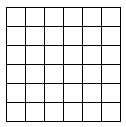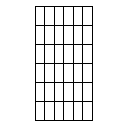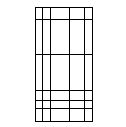An example of a uniform full grid is:

`[X,Y] = meshgrid([1 2 3],[3 6 9 12])`
```X = 1 2 3 1 2 3 1 2 3 1 2 3 Y = 3 3 3 6 6 6 9 9 9 12 12 12```

#### Compact Grid

Explicitly defining every point in a grid can consume a lot of memory when you are dealing with large grids. The compact grid representation is a way to dispense with the memory overhead of a full grid. The compact grid representation stores only grid vectors (one for each dimension) instead of the full grid. Together, the grid vectors implicitly define the grid. In fact, the inputs for `meshgrid` and `ndgrid` are grid vectors, and these functions replicate the grid vectors to form the full grid. The compact grid representation enables you to bypass grid creation and supply the grid vectors directly to the interpolation function.

For example, consider two vectors, `x1 = 1:3` and `x2 = 1:5`. You can think of these vectors as a set of coordinates in the `x1` direction and a set of coordinates in the `x2` direction, like so: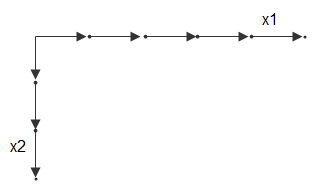Each arrow points to a location. You can use these two vectors to define a set of grid points, where one set of coordinates is given by `x1` and the other set of coordinates is given by `x2`. When the grid vectors are replicated, they form two coordinate arrays that make up the full grid: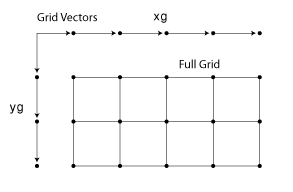Your input grid vectors might be monotonic or nonmonotonic. Monotonic vectors contain values that either increase in that dimension or decrease in that dimension. Conversely, nonmonotonic vectors contain values that fluctuate. If the input grid vector is nonmonotonic, such as `[2 4 6 3 1]`, then `[X1,X2] = ndgrid([2 4 6 3 1])` outputs a nonmonotonic grid. Your grid vectors should be monotonic if you intend to pass the grid to other MATLAB functions. The `sort` function is useful to ensure monotonicity.

#### Default Grid

In some applications, only the values at the grid points are important and not the distances between grid points. For example, most MRI scans gather data that is uniformly spaced in all directions. In cases like this, you can allow the interpolation function to automatically generate a default grid representation to use with the data. To do this, leave out the grid inputs to the interpolation function. When you leave out the grid inputs, the function automatically considers the data to be on a unit-spaced grid. The function creates this unit-spaced grid while it executes, saving you the trouble of creating a grid yourself.

### Example: Temperature Interpolation on 2-D Grid

Consider temperature data collected on a surface at regular 5 cm intervals, extending 20 cm in each direction. Use `meshgrid` to create the full grid.

`[X,Y] = meshgrid(0:5:20)`
```X = 0 5 10 15 20 0 5 10 15 20 0 5 10 15 20 0 5 10 15 20 0 5 10 15 20 Y = 0 0 0 0 0 5 5 5 5 5 10 10 10 10 10 15 15 15 15 15 20 20 20 20 20```

The (x,y) coordinates of each grid point are represented as corresponding elements in the `X` and `Y` matrices. The first grid point is given by `[X(1) Y(1)]`, which is `[0 0]`, the next grid point is given by `[X(2) Y(2)]`, which is ```[0 5]```, and so on.

Now, create a matrix to represent temperature measurements on the grid and then plot the data as a surface.

```T = [1 1 10 1 1; 1 10 10 10 10; 100 100 1000 100 100; 10 10 10 10 1; 1 1 10 1 1]; surf(X,Y,T) view(2)```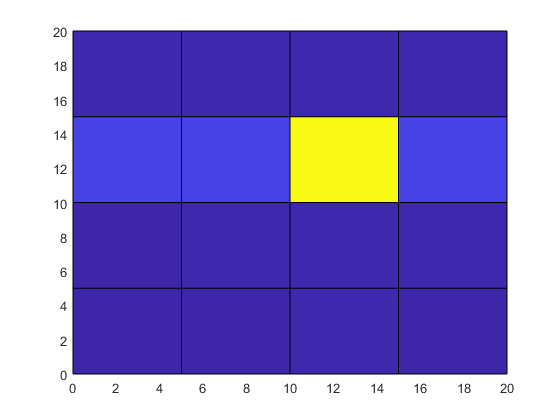Although the temperature at the center grid point is large, its location and influence on surrounding grid points is not apparent from the raw data.

To improve the resolution of the data by a factor of 10, use `interp2` to interpolate the temperature data onto a finer grid that uses 0.5 cm intervals. Use `meshgrid` again to create a finer grid represented by the matrices `Xq` and `Yq`. Then, use `interp2` with the original grid, the temperature data, and the new grid points, and plot the resulting data. By default, `interp2` uses linear interpolation in each dimension.

```[Xq,Yq] = meshgrid(0:0.5:20); Tq = interp2(X,Y,T,Xq,Yq); surf(Xq,Yq,Tq) view(2)```Interpolating the temperature data adds detail to the image and greatly improves the usefulness of the data within the area of measurements.

### Gridded Interpolation Methods

The grid-based interpolation functions and objects in MATLAB offer several different methods for interpolation. When choosing an interpolation method, keep in mind that some require more memory or longer computation time than others. You may need to trade off these resources to achieve the desired smoothness in the result. The following table gives a preview of each interpolation method applied to the same 1-D data, and also provides an overview of the benefits, trade-offs, and requirements for each method.

MethodDescription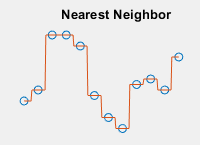The interpolated value at a query point is the value at the nearest sample grid point.

• Discontinuous

• Modest memory requirements

• Fastest computation time

• Requires 2 grid points in each dimension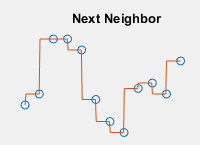The interpolated value at a query point is the value at the next sample grid point.

• Discontinuous

• Same memory requirements and computation time as nearest neighbor

• Available for 1-D interpolation only

• Requires at least 2 grid points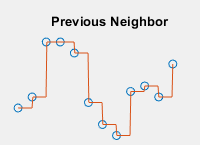The interpolated value at a query point is the value at the previous sample grid point.

• Discontinuous

• Same memory requirements and computation time as nearest neighbor

• Available for 1-D interpolation only

• Requires at least 2 grid points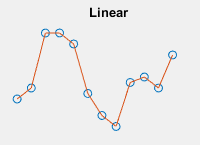The interpolated value at a query point is based on linear interpolation of the values at neighboring grid points in each respective dimension.

• C0 continuous

• Requires more memory and computation time than nearest neighbor

• Requires at least 2 grid points in each dimension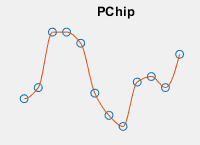The interpolated value at a query point is based on a shape-preserving piece-wise cubic interpolation of the values at neighboring grid points.

• C1 continuous

• Requires more memory and computation time than linear

• Available for 1-D interpolation only

• Requires at least 4 grid points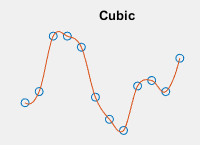The interpolated value at a query point is based on cubic interpolation of the values at neighboring grid points in each respective dimension.

• C1 continuous

• Requires more memory and computation time than linear

• Grid must have uniform spacing, though the spacing in each dimension does not have to be the same

• Requires at least 4 points in each dimension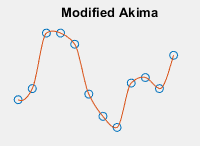The interpolated value at a query point is based on a piecewise function of polynomials with degree at most three evaluated using the values of neighboring grid points in each respective dimension. The Akima formula is modified to avoid overshoots.

• C1 continuous

• Similar memory requirements as spline

• Requires more computation time than cubic, but typically less than spline

• Requires at least 2 grid points in each dimension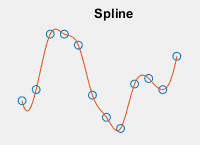The interpolated value at a query point is based on a cubic interpolation of the values at neighboring grid points in each respective dimension.

• C2 continuous

• Requires more memory and computation time than cubic

• Requires at least 4 points in each dimension